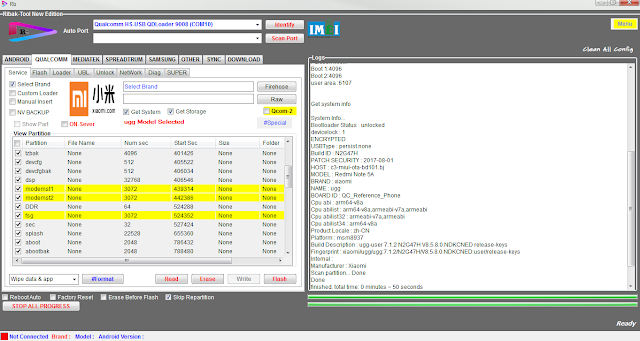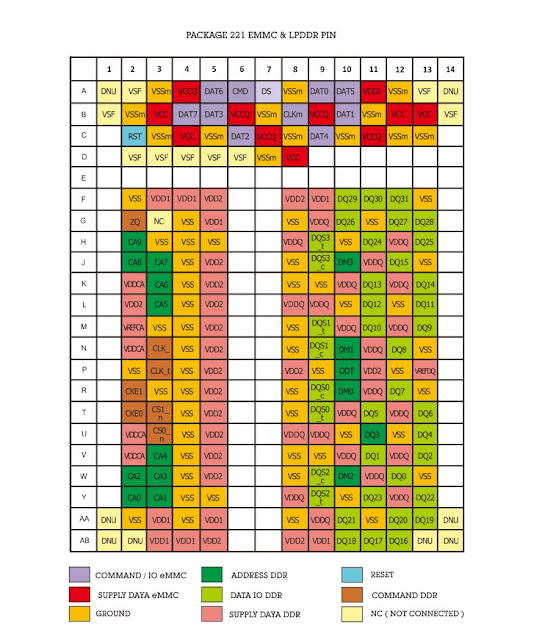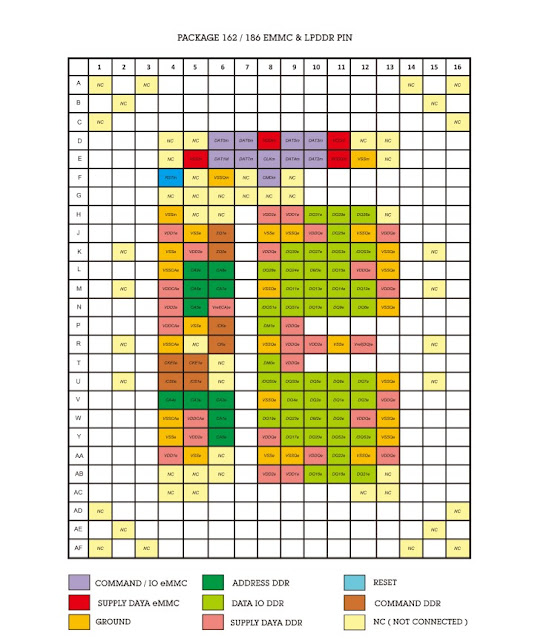Ribak'-Tool-New Inovation Donasi 2OOK+iNCLOUD Vip Member Service Update 2022Ketua Teknisi HP (Official) Ribak-Tool Modul Qualcomm Ribak'S-Tool-New Inovation Donasi 2OOK+iNCLOUD Vip Member Service. .Update Terbaru dan Penambahan fitur dan fungsi yang akan mempermudah pengguna dalam melakukan eksekusi SUPPORT CHIP MTK QUALCOMM SPREADTRUM EXYNOS/SAMSUNG MTK #Super Flash (Flashing) #Read,Write,Erase Partition #Unlock Bootloader #Skip AUTH New Methode #Remove Lock,Frp,pin,Mi Cloud One Click etc QUALCOMM #Super Flash (Flashing) #Read,Write,Erase Partition #Unlock Bootloader #Skip AUTH New Methode #Remove Lock,Frp,pin,Mi Cloud One Click etc Ribak-Tool Modul Mediatek EXYNOS/SAMSUNG #Remove Frp One Click #Remove Frp TEST POINT (MTK & QUALCOMM) #Remove Frp_Send BROWSER(SENAM JARI) #Remove Account Samsung One Click #Unbrick Upgrade ENCOUNTERED AN Issue One Clcik #Repair Baseband One Click etc SPREADTRUM Remove Frp etc ADB #Remove Frp #Bypass Mi Cloud Anti Relock #Sign Out Mi Cloud  #Diag Mode (NO Root) #Diag Mode ( Root) #Remove Frp Samsung OS 10/11 #Sony Anti Th

WAJIB DIKETAHUI !!!

Mohon maaf untuk pengunjung,semua link dinonaktifikan/rusak, dikarenakan kami sedang melakukan pemindahan file ke drive baru,sekaligus perbaikan link yang selalu terjebak di mode iklan dan memperbaharui link yang sudah mati,jika mendesak bisa langsung request pada kolom komentar.

TERIMA KASIH

Persamaan ic eMMC/eMMC (UFS) Update 2022

Ketua Teknisi HP (Official)BGA221

Persamaan ic eMMC/eMMC (UFS) Update 2022.Berikut persamaan ic emmc/ufs yang kami rangkumkan untuk mempermudah dalam proses peragantian.dimana pada postingan sebelumnya kami juga sudah membagikan Persamaan IC PMU-PMI-IC AUDIO-IC BASEBAND dan banyak lagi..

Persamaan ic EMMC/UFS:

MICRON =  JWA60 = HTC 526G         = SONY E2033
MICRON =  JY932 = OPPO A37F
MICRON =  JY941 = G530H       = G531F = G531H
MICRON =  JY950 = NOKIA 3 (TA-1032)
MICRON =  JZ023 = HUAWEI P20 LITE (ANE-LX1) = JKM-LX1
MICRON =  JZ049 = LND-L21 (HL2LNDM)
MICRON =  JZ050 = LND-L21 (HL2LNDM) = XIAOMI REDMI S2
MICRON =  JZ053 = XIAOMI NOTE 6 PRO = XIAOMI MI A2
MICRO     =  JZ100  = A715F
MICRO     =  JZ109 = XIAOMI REDMI NOTE 8
MICRO     =  JZ160  = STK-L21M
MICRO     =  JZ159   = STK-L21M (HL1STKM)

TOSHIBA = TY90HH131625RA = I9300 = SAMSUNG TAB T311
TOSHIPA = TY90HH131635RA = N750
TOSHIBA = TYC0FH121626RA = Y520-U22 = Y330-U01
TOSHIBA = TYDOHH251623RC = HOL-U19
TOSHIBA = TYDOFH221627RA = ALCATEL 7040
TOSHIBA = TYDOGH221640RA = HUAWEI Y635-L21
TOSHIBA = TYDOGH231624RC = I9060
TOSHIBA = THGBMA65A1JBAIR = SONY C2005
TOSHIBA = THGBMAG6A2JBAIR = XIAOMI REDMI 1S
TOSHIBA = THGBMAG7A2JBAIR = HUAWEI ASCEND P7 .
TOSHIBA = TYR01H331667RB = XIAOMI REDMI NOTE 4
TOSHIBA = THGBMBG7D2KBAIL = N7505 = SONY D6633 = HUAWEI MT7-
TL10 = G903F = T705 = HONOR 6 PLUS = MEIZU MX4 PRO = XIAOMI MI 4
= XIAOMI MI NOTE = SAMSUNG T331 = SAMSUNG T337T = G800F =
G750A = MT7-L09
TOSHIBA = THGBMBG8D4KBAIR = G900T
TOSHIBA = THGBMBG7D4KBAIW = I9515
TOSHIBA = THGBMBG7C2KBAIL = G900P
TOSHIBA = THGBMBG6D1KBAIL = G7102 = T113 = T116NU = LG D722 =
LG F430L = T231
TOSHIBA = THGBMFG8C2LBAIL = G610F = G611F
TOSHIBA = THGBMFG6C1LBAIL = SAMSUNG TAB T285
TOSHIBA = THGBMFG7C2LBAIL = A510F = I9300I = I9301I = A710FD =
J700F = J700H = A510FD = G900F = SAMSUNG P555
TOSHIBA = THGBMFG7C1LBAIL = A310F = J510FZ = J710F               = J710F
TOSHIBA = THGBMHG8C4LBAIR = MEIZU MX6
TOSHIBA = THGBMHG9CLBAIR = HUAWEI HONOR NOTE 8
TOSHIBA = THGBM5G7A4JBA4W = I9505
TOSHIBA = THGBM5G7A2JBAIR = G900F
TOSHIBA = THGBM5G6A2JBAIR = SONY D2302
TOSHIBA = THGBM5G8A4JBAIR  = LG OPTIMUS G E975
TOSHIBA = THGBF7G8K4LBATR = G930F = LG G5
TOSHIBA = THGLF2G9J8LBATR = XIAOMI MI 5
TOSHIBA = THGAF4T0N8LBAIR = HUAWEI MATE 10 PRO
TOSHIBA = THGAF8G9T43BAIR = A515F
TOSHIBA = THGAF8T0T43BATR = HUAWEI HONOR MAGIC 2
TOSHIBA = THGAF8T0T43BAIR = HUAWEI MATE 20 PRO = HUAWEI
NOVA 3 = A515F

KINGSTON = KE4CN2L2SA5H2A = HUAWEI Y221-U22
KINGSTON = 08EMCP04-EL3BT227 = ITEL A12
KINGSTON = 08EMCP08-EL3DT227 = TECNO W3
KINGSTON = 08EMCP08-EL2BV100 = TECNO Y4
KINGSTON = 08EMCP08-EL3AV100 = TECNO L5
KINGSTON = 16EMCP08-EL3CV100-A = INFINIX X554
KINGSTON = 08EMCP08-EL3BT227 = TECNO W3 LTE = ITEL IT1556
PLUS
KINGSTON = 16EMCP08-EL3BT527 = TECNO W4S = TECNO L8 LITE
KINGSTON = 16EMCP16-EL3DT527 = TECNO C7 = INFINIX X601

KINGSTON = 16EMCP08-NL3DT527 = TECNO K7 = INFINIX X5010
KINGSTON = 08EMCP04-NL3DT227 = ITEL P12 = ITEL IT1508 = ITEL P13
MINGSTON = 08EMCP04-NL2DT227 = TECNO Y2 = ITEL IT1408
KINGSTON = 08EMCP08-NL3DT227 = TECNO F2
KINGSTON = 04EMCP04-NL2DM627 = SICO MARTPHONE MORE 2

SANDISK = SDIN7DU2-8G = I8190 = I8200 = I9200 = I9070 = I9195 = S7275
= T111 = T230

SANDISK = SDI     BDG4-32G = M205F
SANDISK = SDINBDD4-64G= RNE-L21
SANDISK = SDIN7DP4-16G = HTC ONE MAX
SANDISK = SD7DP28C-4G = HUAWEI Y625-U32 = HUAWEI G730-U10
SANDISK = SDINADF4-64G = LG H961           = LG H960
SANDISK = SDINADF4-32G = HUAWEI HONOR V9
SANDISK = SDIN8DE1-8G = HUAWEI HONOR 4X (CHE2-L22) = CHM-U01
SANDISK = SDIN8DE2-8G = HTC D816 (A5-UL)
SANDISK = SDIN8DE4-16G = HTC ONE E8 (MEC-DUGL)
SANDISK = SD5DH24C-4G = S5301 = S6810
SANDISK = SD5DH24A-4G = S6802
SANDISK = SDIN9DW4-16G = LG G3 = LG D855 = MT7-L09
SANDISK = SDIN9DS2-16G = HTC A16-DWGL(D630N)
SANDISK = SDIN8DE2-4G = HTC ( A11-CHL )
SANDISK = SDIN5C2-8G = I8530
SANDISK = SDIN5C2-32G = SONY LT26ii
SANDISK = SDIN4C2-8G = I9000
SANDISK = SDINBDA4-128G = HUAWEI HONOR 8X
SANDISK= SD7DP24C-4G = HUAWEI Y330-U01

SKHYNIX = H9TP18A8JDMC = I          JOO NOTE = LENOVO A3500-HV
SKHYNIX = H9TP32A4GDDC = J110H = J100H = HUAWEI Y336-U02 =
HUAWEI Y360-U61 = G318HZ = G350E
SKHYNIX = H9TP32A4GDBC = HUAWEI Y511-U30
SKHYNIX = H9TP32A8JDAC = SONY C2305 = HUAWEI G730-U10 = HTC
D616 = ZTE V967S
SKHYNIX = H9TP32A8JDMC = LENOVO A820
SKHYNIX = H9TP32A8JDBC = HTC D816W = HTC D516
SKHYNIX = H9TP32A8JDCC = WIKO RAINBOW LITE 4G
SKHYNIX = H9TP32A4GDCC = Y600-U20 = LENOVO A319 = Y330-U01 =
Y530-U00 = Y520-U22 = LG D290N
SKHYNIX = H9TP65A8JDAC = OPPO 1201 = TAB HUAWEI S7-721G = HTC
D616 = WIKO RAINBOW 4G
SKHYNIX = H9TP64A8JDMC = Y635-L02 = FLY FS502
SKHYNIX = H9TQ64A8GTMC = J200H = LUA-U22 = G531F = Y560-U02 =
SCL-L21 (QUALCOMM) = CU         -L21 = LENOVO A2020A40 = HTC 626PH =
CU   -U29 = ZTE BLADE L5
SKHYNIX = H9TQ64A8GTAC = J106H = J200G
SKHYNIX = H9TQ32A6BTMC = J110F
SKHYNIX = H9TQ53ACLTMC  = XIAOMI REDMI 8 (M1908C3IC)
SKHYNIX = H9TQ32A4GTMC = NOKIA 8110 ( TA-1059 )
SKHYNIX = H9TQ52ACLTMC = REALME 3 ( RMX1821 ) = XIAOMI MI A1 =
HUAWEI HONOR 6X = XIAOMI MI NOTE 4 = HONOR 9N ( LLD-AL20 )
SKHYNIX = H9TQ64A8GTCC = HUAWEI CRO-L22 = J120F
SKHYNIX = H9TQ64A8JTMC = G531F
SKHYNIX = H9TQ64ABJTMC = LENOVO S60 = SONY E2303
SKHYNIX = H9TQ64AAETAC = J500F = G532F = J320H
SKHYNIX = H9TQ17ABJTMC = TECNO C9 = WIKO RIDGE 4G =
TIT-U02 = KII-L21 = HTC D828W = TECNO BOOM J8 = SONY E5303 =
MICROMAX Q450 = TAG-L21
SKHYNIX = H9TQ17A8GTMC = TAB HUAWEI T1-701U
SKHYNIX = H9TQ17A8JTMC = HTC D820pi (MTK)
SKHYNIX = H9TQ17ADFTAC = HONOR 8 LITE (PRA-LA1) = SONY
F3212 = XIAOMI REDMI 6A
SKHYNIX = H9TQ17ADFTMC = HUAWEI HONOR 5X
SKHYNIX = H9TQ17ABJTBC = HUAWEI MYA-L22 = XIAOMI NOTE 5A =
DRA-LX2 = HUAWEI HONOR ( DUA-L22 )
SKHYNIX = H9TQ17ABJTCC = ATU-L31
SKHYNIX = H9TQ18ABJTMC = HUAWEI P7-L00 = OPPO R5 = HUAWEI G7-
L11
SKHYNIX = H9TQ64A6BTMC = J105H
SKHYNIX = H9TQ26ADFTAC = HUAWEI GR5 2017 (BLL-L21) =
LENOVO K53A48 = OPPO A73 ( CPH1725 ) = OPPO A83 ( CPH1729 ) =
XIAOMI REDMI NOTE 4
SKHYNIX = H9TQ27ADFTMC = LD          -L21 (HL1LDNM) = XIAOMI REDMI 7 =
DUB-LX1
SKHYNIX = H9TQ26ADFTBC = INFINIX X522 = NOKIA 6 ( TA-1021 )
SKHYNIX = H9TQ26ADFTMC = HUAWEI RIO-L01
SKHYNIX = H9TQ26ACLTMC = OPPO F5
SKHYNIX = H9HP52ACPMAD = REALME 3 PRO ( RMX1851 ) = OPPO F9
PRO ( CPH1823 )
SKHYNIX = H9HQ53AECMMD  =  REALME 5 PRO ( RMX1971 ) = XIAOMI
MI 9 LITE
SKHYNIX = H9HQ16AFAMMD = OPPO A9 2020 ( CPH1937 )
SKHYNIX = H9HQ53ACPMMD  = VIVO Y19
SKHYNIX = H9HP52ACPMMD = OPPO F7 ( CPH1819 )  = NOKIA TA-1119
SKHYNIX = H9HP52AECMMD  = OPPO F11 PRO ( CPH1969 ) = XIAOMI
REDMI NOTE 7
SKHYNIX = H9HP16AECMMD  = OPPO F11 ( CPH1911 ) = OPPO F11 PRO
( CPH1969 )
SKHYNIX = H9DP32A4JJAC = LENOVO A586
SKHYNIX = H26M54003EMR = HTC M8
SKHYNIX = H26T87001CMR = HUAWEI INE-LX1R = SNE-AL00
(HL1SNEAL00M) = JS      -L22
SKHYNIX = H26M52103FMR = HTC D826Y (A52-DTUL) = HTC ONE E9 (
A53ML-DTUL )
SKHYNIX = H26M41103HPR = HTC A5-DUG
SKHYNIX = H26M64003DQR = HTC M7-UL
SKHYNIX = H26M64208EMR = WAS-LX1A
SKHYNIX = H26M42003GMR = XIAOMI REDMI NOTE
SKHYNIX = H26M78208CMR = HUAWEI HONOR 9 LITE = SONY F8332BGA169/153

SAMSUNG = KMJ5X000WM-B413 = NOKIA XL
SAMSUNG = KMJ5U000WA-B409 = I8262D
SAMSUNG = KMJ5U000WM-B409 = S6312
SAMSUNG = KMJJS000WA-B409 = S7562
SAMSUNG = KMJS000VM-B309 = LENOVO S890
SAMSUNG = KMN5X000ZM-B209 = G313H = G313HZ = HTC D310U =
G313HU = G350E = G130E
SAMSUNG = KMN5X000ZA-B209 = S7262
SAMSUNG = KMN5U000ZM-B203 = LENOVO A369I = S7262
SAMSUNG = KMN5U000FM-B203 = S6810P = S5303 = S6810
SAMSUNG = KMNJS000ZM-B205 = LENOVO P700I
SAMSUNG = KMQNW000SM-B316 = i9060i = G360H = G5308W = G360FY
SAMSUNG = KMQN1000SM-B316 = G361H = XIAOMI REDMI 2A
SAMSUNG = KMQN10006B-B318 = G532F = J320F
SAMSUNG = KMQN10006M-B318 = J500H = J320H = J210F = J500F                =
J500G
SAMSUNG = KMQX10013M-B419 = XIAOMI REDMI 4A = LG MP260 = LG
PM450
SAMSUNG = KMQX60013M-B419 = J400F
SAMSUNG = KMQ820013M-B419 = TECNO J8 = LENOVO P1MA40 =
TECNO L8 PLUS = INFINIX X521 = LENOVO A6020A46 = INFINIX X600
LTE = LENOVO K32C36 = TECNO C9
SAMSUNG = KMQ8X000SA-B414 = G530F = INFINIX X551 = LENOVO TAB
A7-30HC
SAMSUNG = KMQ310006A-B419 = A300FU = J500F
SAMSUNG = KMQ310013B-B419 = J510FN = J3110 = J510GN = J727V
SAMSUNG = KMQ31000SM-B417 = A300H
SAMSUNG = KMQ310013M-B419 = LG K43 = SAMSUNG A7000 = G570F =
SONY F3111 = G550FY
SAMSUNG = KMQ72000SM-B316 = LENOVO A2010-A = ZTE BLADE A510
SAMSUNG = KMQ7X000SA-B315 = LENOVO A6000 = HTC D620G =
TECNO L6 = LENOVO A5000 = LENOVO A6010 = ZTE BLADE L3 = ZTE
SAMSUNG = KMQE10013M-B318 = TECNO CX = TECNO L9 Plus =
TECNO K9 = NOKIA 3 (TA 1032) = XIAOMI REDMI 4A = INFINIX X556 =
INFINIX X572 = SONY G3312 = CAM-L21
SAMSUNG = KMQE6006M-B318 = J327T1
SAMSUNG = KMQE60013M-B318 = J330F = HUAWEI Y5 PRIME (DRA-
LX2) = J400F = INFINIX X608 = OPPO A83 ( CPH1729 ) = OPPO A3S =
REALME C1 ( RMX1811 ) = ATU-L21 = XIAOMI REDMI 6A
SAMSUNG = KMGP6001BM-B514 = OPPO A7 ( CPH1901 ) = XIAOMI
REDMI 7 = DUB-LX1
SAMSUNG = KMGD6001BM-B421 = REALME U1 ( RMX1831 ) = A207F =
HTC DESIRE 12 PLUS = INFINIX X623 = HUAWEI (LD         -L01)
SAMSUNG = KMGX6001BM-B514 = J415F = A605F
SAMSUNG = KMQD60013M-B318 = A107F = XIAOMI REDMI 8A = AM                  -
LX9 = OPPO A1K ( CPH1923 ) = MRD-LX1F = XIAOMI REDMI 7A
SAMSUNG = KMQE60006M-B318 = J250F
SAMSUNG = KMS5U000KM-B308 = S5282
SAMSUNG = KMSJS000JM-B308 = S5300
SAMSUNG = KMSJS000KM-B308 = HUAWEI U8825D
SAMSUNG = KMFN10012A-B214 = J120G = J200F = J106F
SAMSUNG = KMFN10012M-B214 = G531H = J111F = J120H = J200GU =
TECNO F1BGA162/186

SAMSUNG = KMF820012M-B305 = TECNO W5 LITE = TECNO C8 =
TECNO L8 = TECNO W4 = TECNO J5 = INFINIX X600 = INFINIX X554 =
TAB LENOVO A7-30HC = LENOVO TAB (TB3-710L)  = INFINIX X510
SAMSUNG = KMFE10012M-B214 = TECNO W5 = TECNO WX4 = ITEL P51
= INFINIX X557 = LENOVO TAB (TB3-710I)
SAMSUNG = KMFE60012M-B214 = TECNO K7 = INFINIX X559 = J410F =
DRA-LX5  = TAB LENOVO A8-50F
SAMSUNG = KMFNX0012M-B214 = LENOVO A1000 = TECNO W3 =
TECNO WX3 = ITEL IT1556 = TECNO W2 = LENOVO A1010A20
SAMSUNG = KMFNX0007M-B214 = J105B = J105H
SAMSUNG = KMFN60012M-B214 = ITEL S12 = HUAWEI CRO-U00 =
NOKIA 2 (TA-1029) = J260F = NOKIA 1 ( TA-1047 ) = NOKIA 1 PLUS ( TA-
1130 )
SAMSUNG = KMF720012M-B214 = LENOVO A1000
SAMSUNG = KMK5X000YM-B314 = G355H = S7580 = S7582
SAMSUNG = KMK5U000VM-B309 = S7270 = S7272
SAMSUNG = KMK7X000VM-B314 = LENOVO A606 = LENOVO A3300-HV =
LENOVO A536 = LENOVO TAB A3300-GV
SAMSUNG = KMFJ20007M-B214 = J100F
SAMSUNG = KMF310012M-B305 = OPPO A33W
SAMSUNG = KMK8V000VM-B410 = LENOVO S6000-H
SAMSUNG = KMK8X000VM-B214 = LENOVO TAB (A5500-HV)
SAMSUNG = KMK7U000VM-B309 = I8552
SAMSUNG = KMK8X000VM-B412 = LENOVO S850
SAMSUNG = KMK8U000VM-B410 = TAB LENOVO A3000-H = TAB LENOVO
A3500-HV
SAMSUNG = KMKUS000VM-B410 = HTC 608T
SAMSUNG = KMVYL000LM-B503 = I9100 = N7000
SAMSUNG = KMVTU000LM-B503 = I9300
SAMSUNG = KMV2W000LM-B506 = N900
SAMSUNG = KMV3W000LM-B310 = I9500 = N7100 = SM-C101
SAMSUNG = KMR310001M-B611 = E700H = SONY E5333 = HUAWEI ALE-
L21 = A500F = E700F = A700FD = A500G = A700H = SONY E5533 =
HUAWEI KIW-L21
SAMSUNG = KMR31000BM-B611 = OPPO R7
SAMSUNG = KMR4Z0001A-B803 = MEIZU M1 NOTE
SAMSUNG = KMR820001M-B609 = HTC D820 (MTK) = LENOVO P70 =
INFINIX X509
SAMSUNG = KMRX1000BM-B614 = HUAWEI Y7 PRIME (TRT-L21A) =
LENOVO K53A48 = SAMSUNG G5700 = HTC X10U (E66-DUGL = OPPO
A57 (CPH1803)) = INFINIX X522 = OPPO F1S = INFINIX X555 = NOKIA 6
(TA-1033) = LENOVO VIBE K6 PLUS = G615F
SAMSUNG = KMRC10014M-B809 = SAMSUNG C7000 = HTC DESIRE 10
PRO
(A56DJ_PRO_DUGL) = OPPO R9 = VIVO X6 PLUS = SAMSUNG C701F
SAMSUNG = KMRD60014M-B512 = OPPO A5 ( CPH1809 )
SAMSUNG = KMRX60014M-B614 = OPPO F5
SAMSUNG = KMRH60014A-B614 = J810G = J610F = XIAOMI MI A1
SAMSUNG = KMR8X0001M-B608 = INFINIX X506 = HUAWEI ASCEND G7
SAMSUNG = KMRX10014M-B614 = SAMSUNG C5000 = VIVO X6 = G615F =
OPPO F1S
SAMSUNG = KMRE1000BM-B512 = VNS-L31
SAMSUNG = KMR210008M-A805 = N910P
SAMSUNG = KMR21000BM-B809 = HTC D728W (A50CML-DTUL)  = ASUS
Z00UD = HTC D628H
SAMSUNG = KMRV50014M-B809 = YOTA YOTAPHONE 3
SAMSUNG = KML7U000HM-B505 = I8262
SAMSUNG = KLMAG2GEND-B032 = G800F
SAMSUNG = KLMAG1JENB-B031 = J710F = A320F = J730F = J700H =
J737A
SAMSUNG = KLMAG1JETD-B041 = J701F = M105G = A260G = J337V
SAMSUNG = KLMAG2GEAC-B001 = SONY D6503 = HTC ONE E8 (MEC-
UL) = HTC M910N = SONY C6903 = HUAWEI HONOR 6 (H60-L04) =
LENOVO K910L
SAMSUNG = KLMAG2WEPD-B031 = INFINIX X552
SAMSUNG = KLMAG2WE4A-A002 = SAMSUNG TAB T211
SAMSUNG = KLMAG2GEAC-B002 = N9005 = I317
SAMSUNG = KLMAG2WEMB-B031 = HTC D820 (A51-DTUL) = MEIZU MX4
SAMSUNG = KLMAG4FEAB-B002 = I9505
SAMSUNG = KLMCG4JENB-B041 = TECNO PHANTOM 6 PLUS = HUAWEI
P9
SAMSUNG = KLMCG2UCTA-B041 = A307FN = A305F = A750F = A405F
SAMSUNG = KLMCG2KETM-B041 = J730F
SAMSUNG = KLMDG4UCTA-B041 = HUAWEI HONOR 7X = A307G =
A750F
SAMSUNG = KLMBG4WEBC-B031 = SONY D6616 = SAMSUNG P605
SAMSUNG = KLMBG4GEAC-B001 = G906S
SAMSUNG = KLMBG2JENB-B041 = A720F
SAMSUNG = KLMBG2JETD-B041 = A730F = J530F = A205F = A105F =
A750FN = A305N = A102U = A202F = M105F = J720F
SAMSUNG = KLMBG4GENO-B031 = HUAWEI MATE 8
SAMSUNG = KLMBG4GEND-B031 = HUAWEI MATE 8 (NXT-L29) = HTC
X9U (E56ML-DTUL) = LG H955 = SONY E6833
SAMSUNG = KLM8G1WEMB-B031 = G7102 = T211 = G7105 = T231 =
HUAWEI G750-U10
SAMSUNG = KLM8G1WEPD-B031 = HTC D626N (A32EUL) = HTC D626S
(A32EWHL)
SAMSUNG = KLM8G1WE4A-A001 = I9105P = I9152 = I9082 = I9100G =
SAMSUNG T211
SAMSUNG = KLM8G1GEAC-B001 = SONY D5103 = SONY D5303 = SONY
D2533 = SONY D5316 = SONY D2303
SAMSUNG = KLM8G2FE3B-B001 = SONY C5303 = SAMSUNG P3310
SAMSUNG = KLM4G1YEMD-B012 = I8160
SAMSUNG = KLUCG8G1BD-E0B1 = G925F = G925I
SAMSUNG = KLUCG4J1ED-B0C1 = G950F = N950F = HUAWEI MATE 10 =
MEIZU PRO 7 PLUS = A505F = G975X = A515F
SAMSUNG = KLUCG4J1EB-B0B1 = HUAWEI MATE 9
SAMSUNG = KLUCG4J1CB-B0B1 = G955F
SAMSUNG = KLUBG4G1BD-E0B1 = G920F = G920I
SAMSUNG = KLUBG4G1CE-B0B1 = G935F
SAMSUNG = KLUDG4U1EA-B0C1 = VIVO IQOO = HUAWEI MATE 20 =
HUAWEI HONOR NOTE 10 = XIAOMI MI 9 = VIVO NEX DUAL DISPLAY =
A515F
SAMSUNG = KLUDG4UHDB-B2D1 = XIAOMI MI 10
SAMSUNG = KLUEG8U1EA-B0C1 = HUAWEI MATE RS
SAMSUNG = K4P8G304EQ-AGC2 = SONY D2502
SAMSUNG = KM3V6001CM-B705 = OPPO FIND X = XIAOMI MI NOTE 3 =
OPPO F7 PRO ( CPH1821 ) = REALME 3 PRO ( RMX1851 ) = OPPO F11
PRO (CPH1969) = VIVO V15 PRO ( 1818 ) = M405F
SAMSUNG = KM3H6001CA-B515 = SAMSUNG A6060 = A606Y
SAMSUNG = KM8V7001JA-B813 = VIVO V17 = A805F
SAMSUNG = KM5H7001DM-B424 = OPPO A5 2020 ( CPH1933 )
SAMSUNG = KM5V7001DM-B621 = OPPO A5 2020 ( CPH1933 )  = OPPO
A9 2020 ( CPH1937 )
SAMSUNG = KM2V7001CM-B706 = VIVO V15 PRO (QUALCOMM) = VIVO
Z1 PRO ( 1951 ) = A705FN = A707F = XIAOMI REDMI NOTE 8 PRO
SAMSUNG = KMDH6001DM-B422 = OPPO R11
SAMSUNG = KMDH6001DA-B422 = VIVO U10 ( 1916 )
SAMSUNG = KMDD60018M-B320 = OPPO A12 (CPH2083)
NCEPNA6M4-0808 = ITEL A16 PLUS